Suppose that each training class is represented by a prototype (or mean) vector: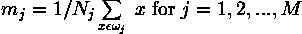where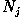is the number of training pattern vectors from class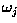. In the example classification problem given above,and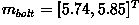as shown in Figure 2.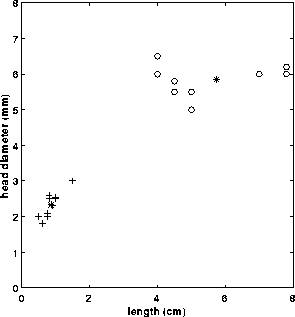Figure 2 Feature space: + sewing needles, o bolts, * class mean

Based on this, we can assign any given patternto the class of its closest prototype by determining its proximity to each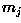. If Euclidean distance is our measure of proximity, then the distance to the prototype is given by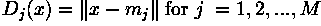It is not difficult to show that this is equivalent to computingand assignto classif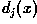yields the largest value.

Other reference:
Mar 24, 2012 gmail 附件讲义7.3节有minimum distance classifier的英文描述